## Example Questions

### Example Question #1 : Intersecting Lines & Angles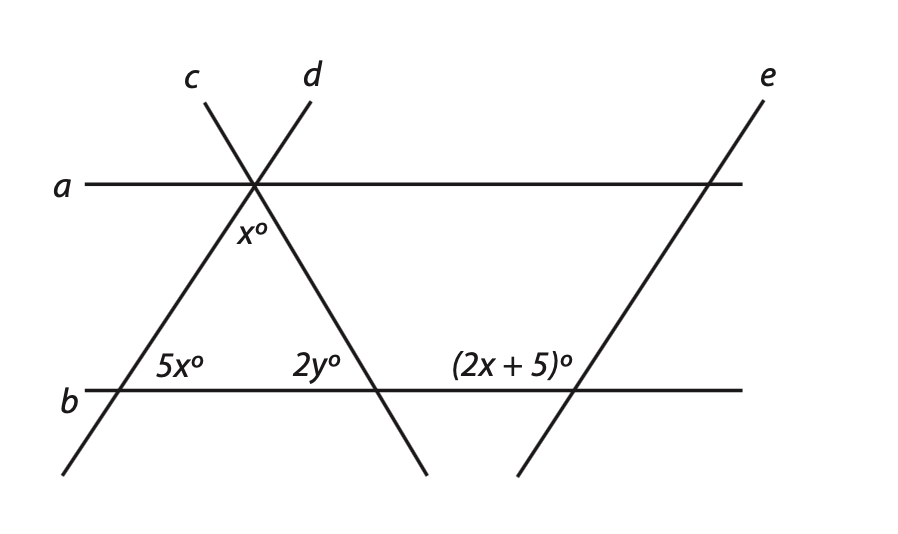In the figure above, line a is parallel to line b and line d is parallel to line e. What is the value of y, in degrees?

15

25

10

30

15

Explanation:

Intersecting and parallel lines show up in many different geometric figures: parallelograms, trapezoids, squares, etc. Anytime you see these in a question, you have to properly leverage the essential properties of supplementary and vertical angles. On this problem, the fastest way to find y is to realize that 5x in the bottom left corner is supplementary to 2x + 5 in the bottom right (because of the intersection of two parallel lines). Therefore, 5x + 2x + 5 = 180 and x = 25. Once you have that information, you can use the fact that the sum of the interior angles of a triangle is 180 and see that x + 5x + 2y = 180 . Putting in 25 for x you see that 25+125+2y =180 and 2y =30. The correct answer is 15.

### Example Question #1 : Intersecting Lines & Angles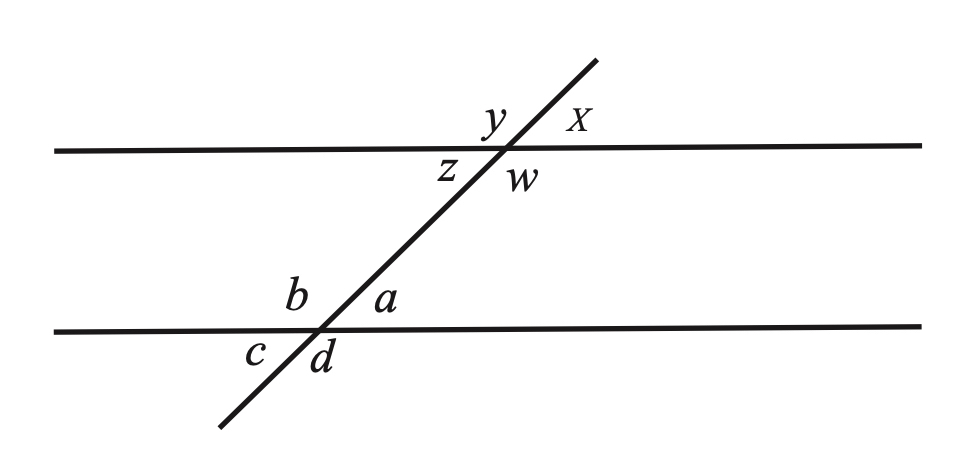In the figure above,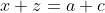. Which of the following must be true?

I.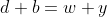II. The two horizontal lines are parallel.

III.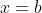I and II only

I and III only

I, II, and III

II and III only

I and II only

Explanation:

Here the SAT gives you a pair of lines with a transversal, but it does not tell you that the lines are parallel - it asks you to prove it. You are told that. Since angle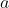and angle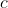are vertical angles and angles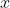and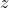are vertical angles, you know that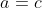and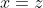. That means you can write your equation as: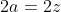, or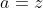Ifthat means that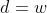as well. A straight line contains 180 degrees, so you know that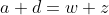. And since, you can conclude thatas well. From here, you can reverse engineer the same sort of equation you solved with the first set of angles. If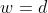and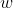and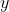are vertical angles and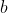and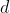are vertical angles, you can conclude that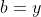. From there you can set up the equation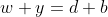. Statement I is true.

In order for the horizontal lines to be parallel, you need to know that either the alternate exterior angles or the alternate interior angles are equal. Since you have already proven that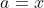, you know also that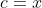. Since you have a pair of alternate exterior angles, the two lines must be parallel. Statement II is also true.

Statement III, however, is not necessarily true. If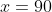then all angles would equal 90. However without that knowledge, you cannot come to any conclusions about the relationship betweenand. Statement III is not necessarily true, so the correct answer is I and II only.

### Example Question #1 : Intersecting Lines & Angles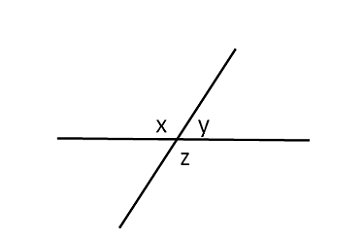Two straight lines intersect to form the angles above. If the measure of angle x is three times the measure of angle y, what is the measure of angle z?

45

120

60

135

135

Explanation:

Since lines x and y will add to a total of 180 degrees, you have two equations to work with:

x + y = 180

x = 3y

This means you can substitute 3y for x in order to solve for y:

3y + y = 180

4y = 180

y = 45

And since z will also sum with y to 180, then z must be 180 - 45 = 135 degrees.

### Example Question #1 : Intersecting Lines & Angles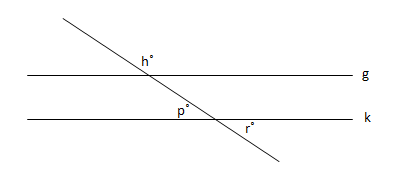In the figure above, if lines g and k are parallel and angle h measures 121 degrees, what is the value of p?

71

49

59

61

59

Explanation:

If h is 121, then the angle immediately below h must be 59, as it is a supplementary angle formed by the diagonal line. Since g and k are parallel, this 59 degree angle must exactly match p as they are alternative interior angles.

### Example Question #2 : Intersecting Lines & Angles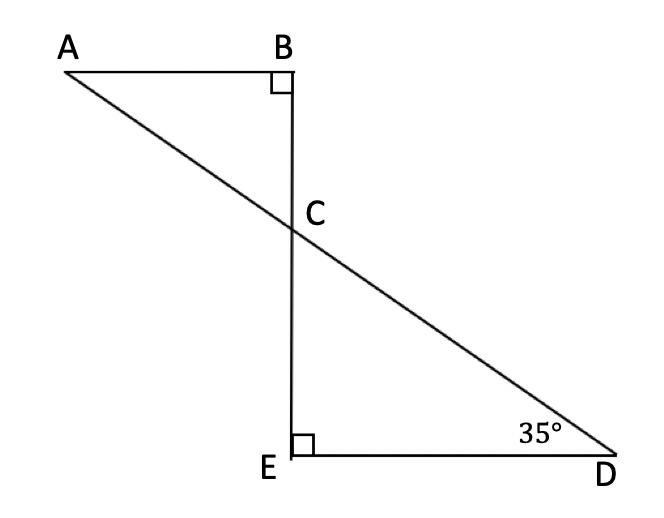In the diagram above, lines AD and BE intersect at point C. What is the measure of angle ACE?

125

135

115

145

125

Explanation:

Two angle rules are very important for this question:

1) The sum of the interior angles of a triangle is always 180. Here, since you have a 90-degree angle (CED) and a 35-degree angle (EDC) in the bottom triangle, you can then conclude that angle ECD must be 55.

2) Supplementary angles, angles that are adjacent to each other when two straight lines intersect, must sum to 180 degrees. If you know that ECD is 55, then ACE as a supplementary angle must form the other 125 degrees for those two angles to sum to 180. Therefore, the correct answer is 125.

### Example Question #4 : Intersecting Lines & Angles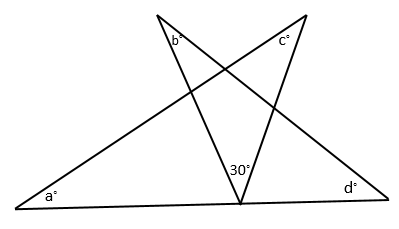What is a + b + c + d?

150

110

120

130

150

Explanation:

An important thing to recognize in this problem is that you're dealing with two intersecting triangles that create external supplementary angles along the straight line on the bottom. To see this, consider the diagram below for which angles x and y have been added: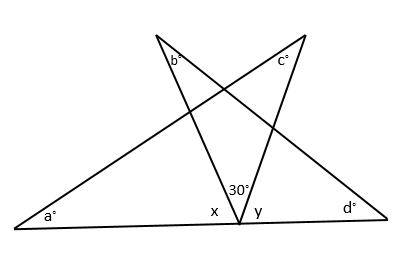Angle y is an external supplementary angle to the triangle beside it so y = a + c. Why? Remember that y is supplementary to the angle beside it (x + 30) and (a + c) is supplementary to that same angle (the sum of interior angles of a triangle = 180.) Therefore y and (a + c) are identical. Anytime you have a straight line drawn off of a triangle you should recognize that the external supplementary angle equals the sum of the two opposite angles.

Using the same logic, you can see that x = b + d in the other intersecting triangle. Since the problem is asking for a + b + c + d, you should recognize that this question is really the same as what is x + y. Why? You can substitute x for b + d and y for a + c in the question stem. Since x + y = 180 - 30 on the straight line along the bottom, the correct answer is 150.

Note that another way to solve this problem involves seeing two large obtuse triangles: one with the angles a, c, and (x+30) and the other with the angles b, d, and (y+30). If you do that, you would have:

a+c+x+30=180, so a+c+x=150

b+d+y+30=180, so b+d+y=150

And you know that x+y+30=180 because x, 30, and y are all angles that make up the 180-degree straight line across the bottom of the figure. So x+y=150.

You can then sum the triangle equations:

a+c+x+b+d+y=150+150=300

And then plug in x+y = 150 and you're left with a+b+c+d=150.

### Example Question #5 : Intersecting Lines & Angles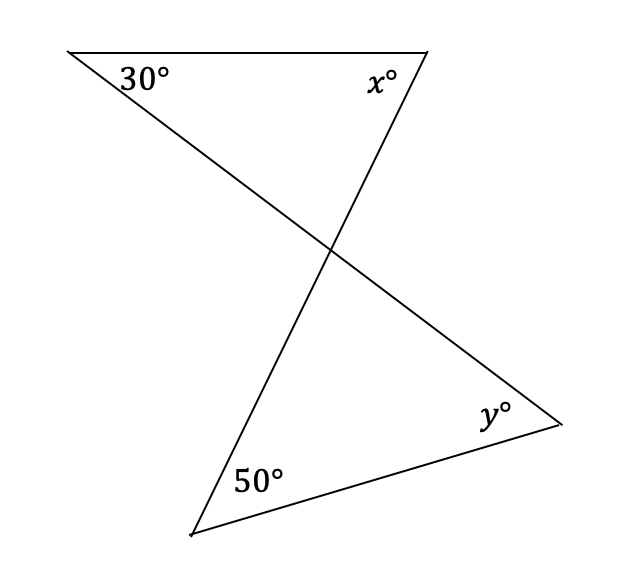In the image above,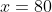. What is the value of60

50

70

80

60

Explanation:

This problem hinges on two important geometry rules:

1) The sum of all interior angles in a triangle is 180. Here you know that in the top triangle you have angles of 30 and 80, meaning that the angle at the point where lines intersect must be 70, since 30+80=110, and the last angle must sum to 180.

2) Vertical angles - angles opposite one another when two straight lines intersect - are congruent. Because you have identified that the angle at the bottom of the triangle at the top is 70, that also means that the top, unlabeled angle of the bottom triangle is 70. That then lets you add 70+50+as the three angles in the bottom triangle, and since they must sum to 180 that means that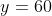.

### Example Question #1 : Intersecting Lines & Angles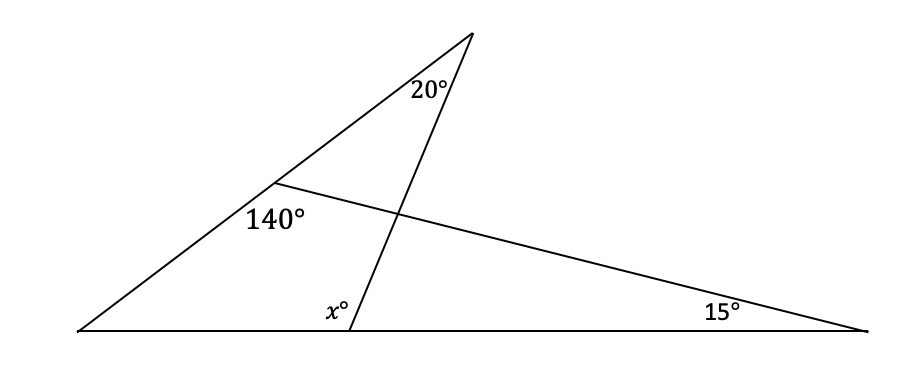NOTE: Figure not drawn to scale.

What is the value ofin the figure above?

135

125

115

150

135

Explanation:

This problem heavily leverages two rules:

1) The sum of the angles in a triangle is 180.

2) Supplementary angles - adjacent angles created when one line intersects another - must sum to 180.

Here you can first leverage the 140-degree angle to fill in that its adjacent neighbor - its supplementary partner - must then be 40. and that gives you two of the three angles in the uppermost triangle: 20 and 40. You can use that to determine that the third angle must then be 120.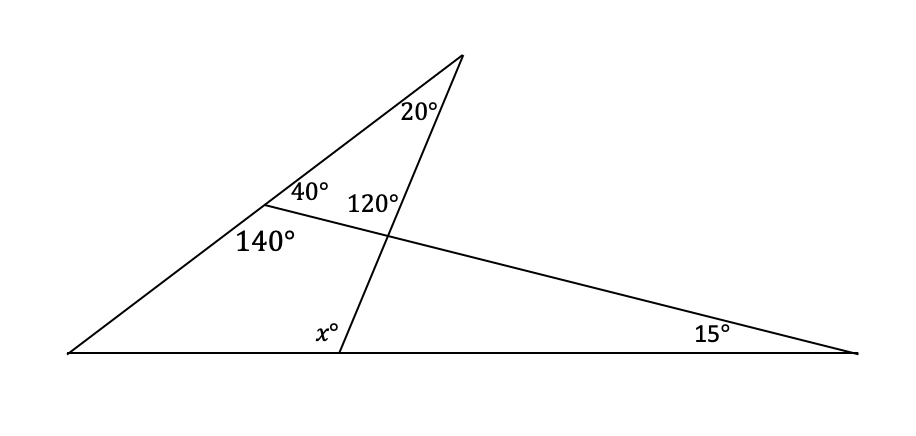From there you should see that the 120-degree angle is a vertical angle, meaning that its opposite will also be 120. And that gives you a second angle in the lower-right triangle. Knowing that you have angles of 15 and 120 means that the third angle of that triangle must be 45.  And since that angle is supplementary to angle x, x must then be 135.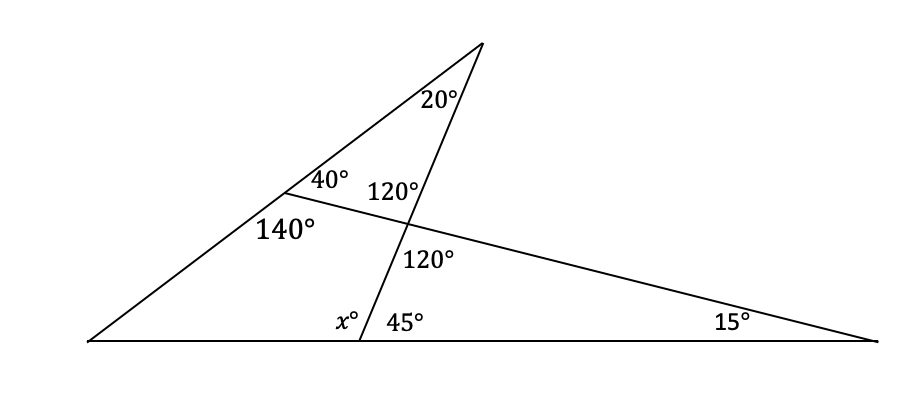### Example Question #1 : Intersecting Lines & Angles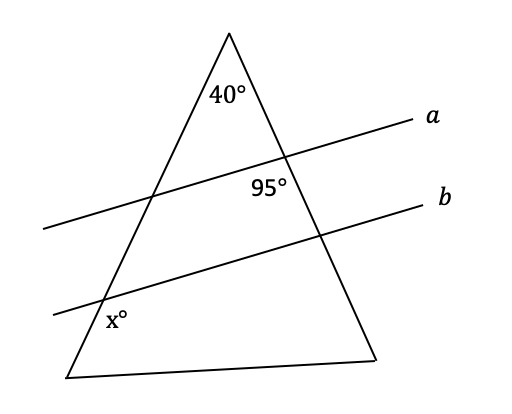In the figure above, linesandare parallel. What is the value of?

105

95

115

125

125

Explanation:

This problem heavily leans on two important lines-and-angles rules:

1) The sum of the three interior angles of a triangle is always 180.

2) Supplementary angles - angles next to each other formed by two lines intersecting - must also sum to 180.

Here you can then determine that the angle next to the 95-degree angle is 85, and since that angle is the lower-right hand angle of the little triangle at the top, you can close out that triangle. With angles of 40 and 85, that means that the lower left hand angle must be 55.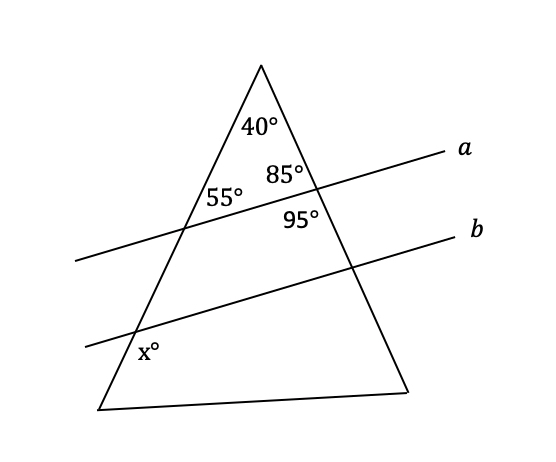From there, you can use the fact that parallel lines will lead to congruent angles. Since linesandare parallel, the angle next towill be 55 degrees, meaning thatwill then be 125.

### Example Question #1 : Intersecting Lines & Angles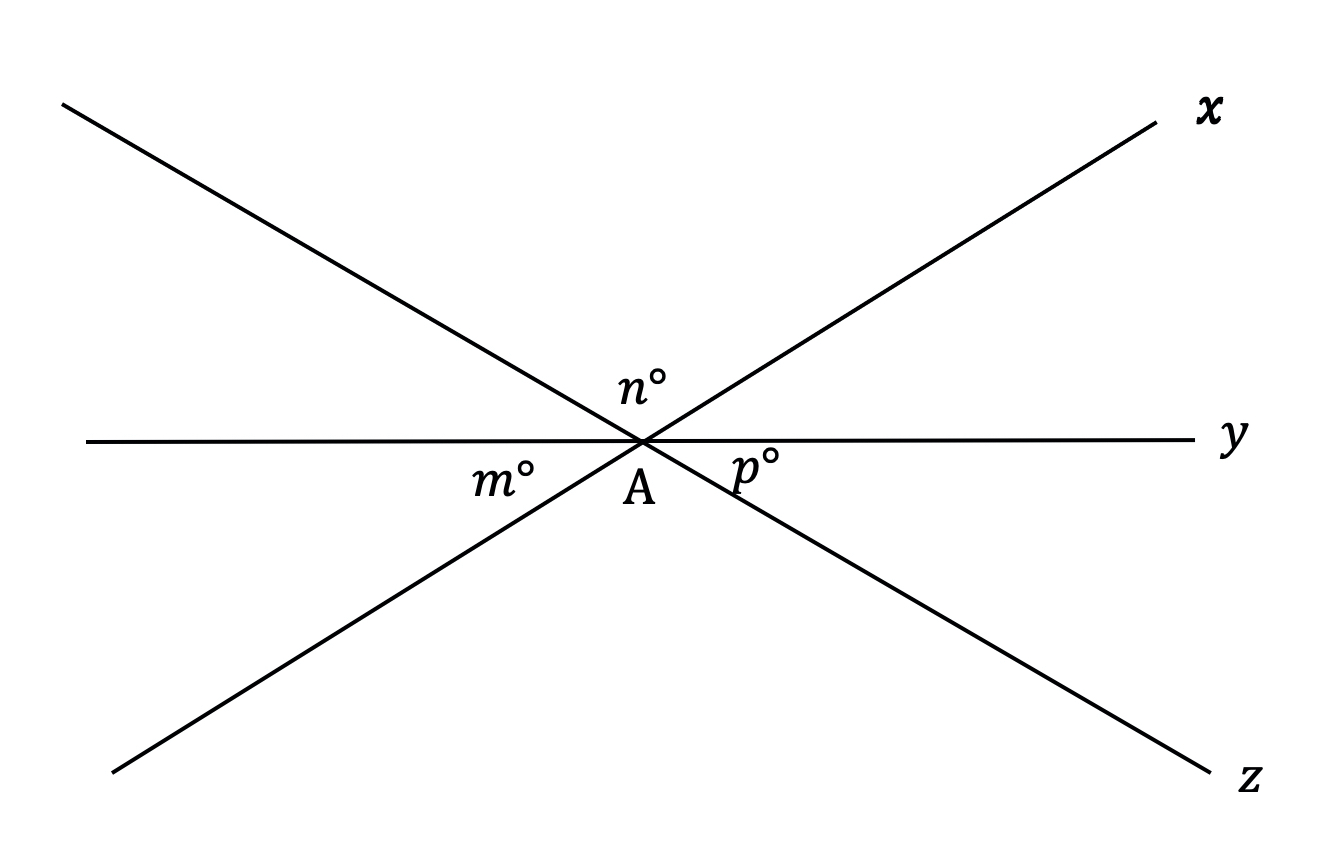In the diagram above, lines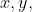andall intersect at point A. If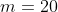and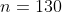, what is the value of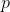?

30

20

40

50

This problem tests two important rules. For one, the angle measure of a straight line is 180. Here if you follow lineyou can see that its angle is broken in to three segments: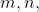and the blank angle between them. Those three angles must sum to 180, so if you already know thatand, then the unlabeled angle between them must equal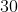so that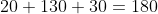.
Next, know that when lines intersect to form angles at a particular point, opposite (vertical) angles are congruent. The angle of measureis directly opposite the angle you just calculated to bedegrees, sohas to beas well.